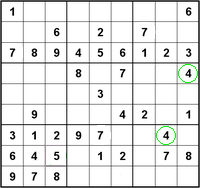# Matrix Vol. 2 Exercises

Want to share your content on R-bloggers? click here if you have a blog, or here if you don't.Answers to the exercises are available here.

Exercise 1

If
```M=matrix(c(1:10),nrow=5,ncol=2, dimnames=list(c('a','b','c','d','e'),c('A','B')))```

What is the value of: `M`

Exercise 2

Consider the matrix `M`,
What is the value of:
`M[1,]`
`M[,1]`
`M[3,2]`
`M['e','A']`

Exercise 3

Consider the matrix `N`
```N=matrix(c(1:9),nrow=3,ncol=3, dimnames=list(c('a','b','c'),c('A','B','C'))) ```
What is the value of: `diag(N)`

Exercise 4

What is the value of: `diag(4,3,3)`
Is matrix ?

Exercise 5

If ```M=matrix(c(1:9),3,3,byrow=T, dimnames=list(c('a','b','c'),c('d','e','f')))```
What is the value of:
`rownames(M)`
`colnames(M)`

Exercise 6

What is the value of:
`upper.tri(M)`
`lower.tri(M)`
`lower.tri(M,diag=T)`

Exercise 7

Consider two matrix,
```M,N ```
`M=matrix(c(1:9),3,3,byrow=T)`
```N=matrix(c(1:9),3,3) ```
What is the value of:

```M*N ```

Exercise 8

Consider two matrix,
```M,N ```
`M=matrix(c(1:9),3,3,byrow=T)`
```N=matrix(c(1:9),3,3) ```
What is the value of:

```M%*%N ```

Exercise 9

Consider two matrix,
```M,N ```
`M=matrix(c(1:9),3,3,byrow=T)`
```N=matrix(c(1:9),3,3) ```

What is the value of:

`(M+N)^2`

Exercise 10
Consider two matrix,
```M,N ```
`M=matrix(c(1:9),3,3,byrow=T)`
```N=matrix(c(1:9),3,3) ```

What is the value of:

`M/N`

Want to practice matrices a bit more? We have more exercise sets on this topic here.

R-bloggers.com offers daily e-mail updates about R news and tutorials about learning R and many other topics. Click here if you're looking to post or find an R/data-science job.
Want to share your content on R-bloggers? click here if you have a blog, or here if you don't.